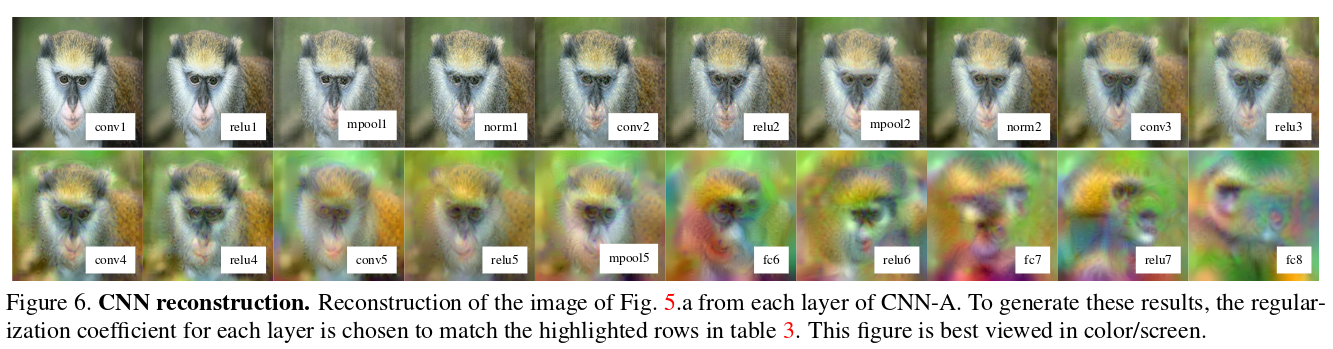Go back to list of posts

#### Nov 23, 2015

Paper title: Understanding Deep Image Representations by Inverting Them

Authors: Aravindh Mahendran, Andrea Vedaldi

Warning: The code in this post is just for illustrative purposes. It isn’t runnable.

## Summary

This paper tries to reconstruct an image from its representation as a way of visualizing its information. The proposed method is applied to representations obtained from CNN, HOG or DSIFT. It tries to find a reconstructed image xr by minimizing the Euclidean distance between the representation of xr and that of the original image. A regularizer is also added to the loss to be minimized to produce more natural looking images. The paper uses SGD with momentum to perform the minimization. Let start focusing on CNNs.

 ```1 2 3 4 5 6 7``` ```# Let's suppose we are using AlexNet and the representation is obtained in its 3rd fully connected (fc) layer. # h is the representation of image x given by the activations of the 3rd fc layer of the CNN. h = cnn_fc3(x) # The paper wants to find one image xr such that cnn_fc3(xr) is close to h xr = reconstruct(h, cnn_fc3) hr = cnn_fc3(xr) sum((hr-h)**2) < epsilon == True # means that the two hs are close. ```

One of the desired properties of CNNs trained for object recognition is that they are invariant to many transformations of the input. That also means that they are not invertible functions. So there are many input images that will produce the same representation.

 ```1 2``` ```# There are many pairs xi and xj such that: np.all(cnn_fc3(xi) == cnn_fc3(xj)) == True ```

Some of these images will probably not even look natural as the following paper on adversarial examples show: Deep Neural Networks are Easily Fooled: High Confidence Predictions for Unrecognizable Images

But the current paper wants only to find natural looking images so it incorporates an image “prior” to the reconstruction method. The way it incorporates the prior is the main novelty of the paper.

The problem of finding the reconstruction is posed as an optimization problem of trying to minimize the Euclidian distance between hr and h:

 `1` ```xr = argmin( lambda x: sum( ((cnn_fc3(x)-h)**2) ) ) ```

But we also need to restrict the possible xr to look natural. So the paper adds also a “prior” as a regularizer to the loss equation:

 `1` ```xr = argmin( lambda x: sum( ((cnn_fc3(x)-h)**2) + regularizer(x) ) ) ```

Actually, the paper uses an equation a bit different from the above to make the guessing of the initial hyper-parameter easier. See section Balancing the different terms for more information.

The regularizer term is defined as the sum of two sub-terms:

 ```1 2 3 4 5 6 7 8 9 10 11 12 13 14 15 16 17 18``` ```# this regularizer is one of the main paper's contribution. def regularizer(x): mult1 = 2.6*1e8 mult2 = 5 return mult1*six_norm(x) + mult2*total_variation(x) def six_norm(x): return sum(x**6) # this is the finite-difference approx. of the total variation def total_variation(x): beta = 2 result = 0.0 # suppose x is properly zero padded. for i in xrange(x.shape): for j in xrange(x.shape): result += ( (x[i,j+1]-x[i,j])**2 + (x[i+1,j]-x[i,j])**2 )**(beta/2) return result ```

The optimization problem is then solved by using SGD with momentum as all the parts of the loss function are differentiable on x (including off course cnn_fc3).

To visualize the representation of HOG and DSIFT the authors first build these methods using the CNN layers so it becomes easy to differentiate on the input. The algorithm then proceeds as before.

## Experiments

The authors tried to reconstruct the images from other layers of the CNN too. The image below, that was extracted from the paper, shows the reconstruction of an image from the representation of different layers of the used CNN.It can be seen that the first layers preserve almost all information of the image and the last layers loses information about the location of the object, for instance, but retain information about some discriminative features of the objects.

## Conclusion

I hope papers like this one ends the myth that Neural Nets are black box algorithms. There are many works that try to visualize and understand what is happening inside them CNNs. Below I list some of them:

The last paper from above has also made available their code: https://github.com/yosinski/deep-visualization-toolbox

I read this paper, because I started reading A Neural Algorithm of Artistic Style and I thought I needed first to read some of its references. So probably my next notes will be about the above paper and the following former work by the same authors: Texture Synthesis Using Convolutional Neural Networks

#### The Fine Print

These notes are similar in spirit to https://twitter.com/hugo_larochelle notes that he announces in his Twitter. The only difference is that from time to time I will also write notes in Jupyter Notebook and insert some small code samples to understand better the concepts exposed in the papers. The code is not meant to reproduce the paper and sometimes may not even be runnable as I pretend not to spend too much time on every paper I read. Sometimes I may wish to dig deeper into a paper and try to reproduce the results, but then I will tag the blog post in a different section of my blog.